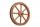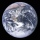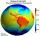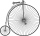# Perimeter + rounding - math problems

#### Number of problems found: 9

• StepsHow many steps you save if you go square estate for diagonal (crosswise), rather than circumvent on the two sides of its perimeter with 307 steps.
• Wheel diameterA 1m diameter wheel rolled along a 100m long track. How many times did it turn?
• The equatorCalculate the length of the equator. Calculate a radius of 6378 km. Round to the nearest thousand. - Procedure and calculation
• GardenHow many steps of 76 cm circumvent square garden with area 1.8 ha?
• Diameter of a cylinderThe diameter of the cylinder is 42 cm. How many times does the cylinder turn on a 66m long track?
• Average speedWhat is the average speed you have to move the way around the world in 80 days? (Path along the equator, round to km/h).
• GardenRectangular garden 31 meters 40 centimeters long and 20m30cm wide adjacent with the shorter side with another (fenced) garden and on one longest side is 1 meter wide gate. How many meters of fence is needed to buy? (Estimate, calculate, verify a test calc
• TableclothsThe restaurant has sixty-two square tablecloths with a side length of 150 cm and 36 rectangular tablecloths with dimensions of 140 cm and 160 cm. A) How many meters of hemming ribbon will be needed if we add 50 cm to each tablecloth? B) The ribbon sale in
• VelocipedesIn the 19th century, bicycles did not have a chain drive, and the pedals were connected directly to the wheel axis. This wheel diameter gradually increased until the so-called high bicycles (velocipedes) with a front wheel diameter of up to 1.5 meters, wh

We apologize, but in this category are not a lot of examples.
Do you have an interesting mathematical word problem that you can't solve it? Submit a math problem, and we can try to solve it.

We will send a solution to your e-mail address. Solved examples are also published here. Please enter the e-mail correctly and check whether you don't have a full mailbox.

Please do not submit problems from current active competitions such as Mathematical Olympiad, correspondence seminars etc...

Do you want to round the number? Perimeter - math word problems. Rounding - math word problems.